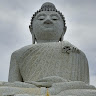# MIDI Pedal Board for guitar multieffect FX-500

A pedal board to send MIDI Program Change to my old guitar multieffect that has a MIDI IN port.

BeginnerProtip606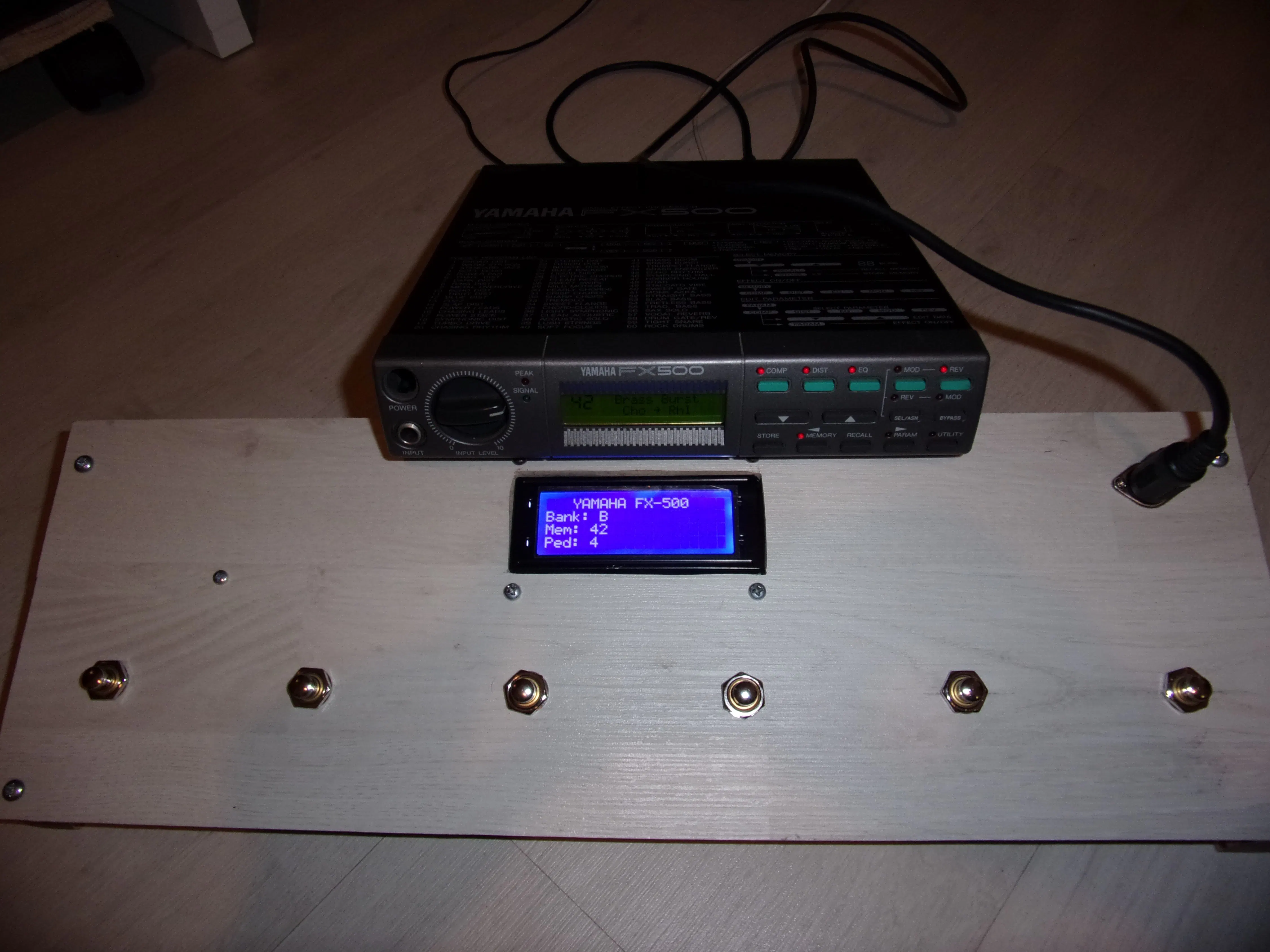## Things used in this project

### Hardware components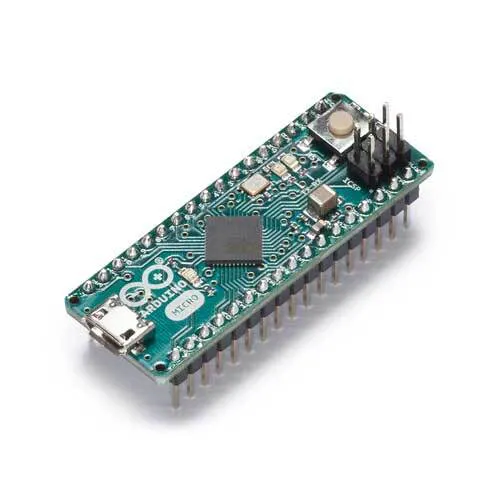Arduino Micro
×1Resistor 10k ohm
×6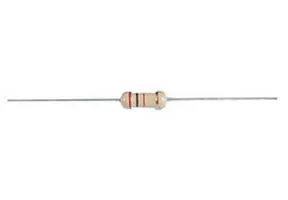Resistor 220 ohm
×2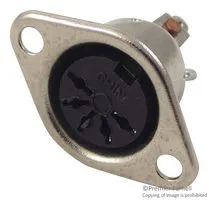DIN Audio / Video Connector, 5 Contacts
×1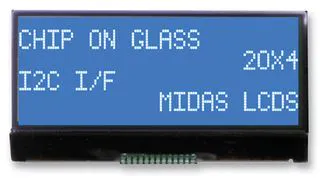Alphanumeric LCD, 20 x 4
×1
 SNAP ACTION SWITCH Z15G1307
×1

### Software apps and online servicesArduino IDE

### Hand tools and fabrication machinesSoldering iron (generic)

## Schematics

### MIDI pedal board schematic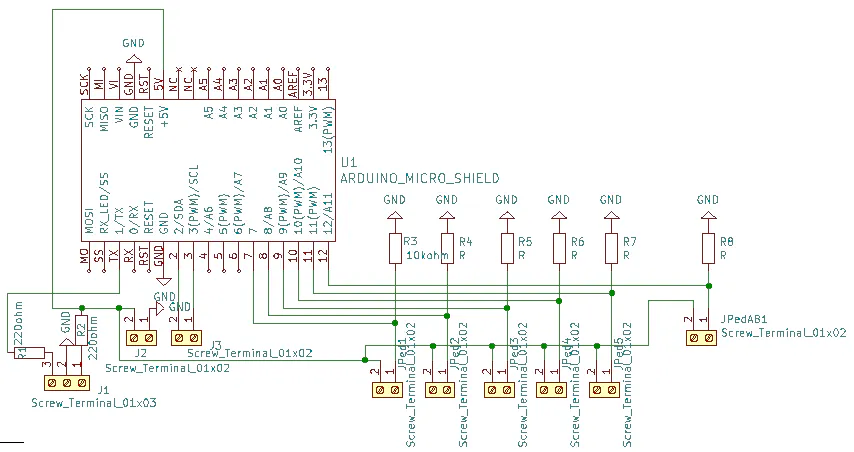## Code

### MIDI pedal board Arduino code

Arduino
```/*
5v-tx-gnd-

pin 7-8-9-10-11-12
pos 1-2-3- 4 -5-AB
*/

#include <MIDI.h>
#include <LiquidCrystal_I2C.h>
LiquidCrystal_I2C lcd(0x27,20,4);  // set the LCD address to 0x27 for a 20 chars and 4 line display

MIDI_CREATE_DEFAULT_INSTANCE();
String bank ="A";
int effA1=10;
int effA2=20;
int effA3=30;
int effA4=40;
int effA5=50;
int effB1=12;
int effB2=22;
int effB3=32;
int effB4=42;
int effB5=52;
int PedBankAB = 0;
int Ped1 = 0;int Ped2 = 0;int Ped3 = 0;int Ped4 = 0;int Ped5 = 0;
int PrCh =0;
int activePedal=1;
long lastDebounceTime=0; //the last time the output pin was toggled
long debounceDelay=800;//the debounce time; increase if the output flickers

void setup() {
// put your setup code here, to run once:
pinMode(7, INPUT);pinMode(8, INPUT);pinMode(9, INPUT);pinMode(10, INPUT);pinMode(11, INPUT);
pinMode(12, INPUT);
MIDI.begin(14);
MIDI.sendProgramChange(effA1, 1);
lcd.init();                      // initialize the lcd
lcd.backlight();
lcd.clear(); //pulisci lo schermo
lcd.setCursor(0, 0); lcd.print("   YAMAHA FX-500");
lcd.setCursor(0, 1); lcd.print("Bank: ");lcd.print(bank);
lcd.setCursor(0, 2); lcd.print("Mem: ");lcd.print(effA1);
lcd.setCursor(0, 3); lcd.print("Ped: ");lcd.print(activePedal);
}

void loop() {
// put your main code here, to run repeatedly:
if((millis()-lastDebounceTime)>debounceDelay) {
// pressing bank (A or B)
if (PedBankAB == HIGH) {
if (bank=="A") {bank="B";
if (activePedal==1) {PrCh=effB1;}
if (activePedal==2) {PrCh=effB2;}
if (activePedal==3) {PrCh=effB3;}
if (activePedal==4) {PrCh=effB4;}
if (activePedal==5) {PrCh=effB5;}
MIDI.sendProgramChange(PrCh, 1);
lastDebounceTime=millis(); //set the current time
}
else {bank="A";
if (activePedal==1) {PrCh=effA1;}
if (activePedal==2) {PrCh=effA2;}
if (activePedal==3) {PrCh=effA3;}
if (activePedal==4) {PrCh=effA4;}
if (activePedal==5) {PrCh=effA5;}
MIDI.sendProgramChange(PrCh, 1);
lastDebounceTime=millis(); //set the current time
}
}
// pressing pedal 1 to 5
if (Ped1 == HIGH) {
activePedal= 1;
if (bank == "A") {PrCh= effA1;}
if (bank == "B") {PrCh= effB1;}
MIDI.sendProgramChange(PrCh, 1);
lastDebounceTime=millis(); //set the current time
}
if (Ped2 == HIGH) {
activePedal= 2;
if (bank == "A") {PrCh= effA2;}
if (bank == "B") {PrCh= effB2;}
MIDI.sendProgramChange(PrCh, 1);
lastDebounceTime=millis(); //set the current time
}
if (Ped3 == HIGH) {
activePedal= 3;
if (bank == "A") {PrCh= effA3;}
if (bank == "B") {PrCh= effB3;}
MIDI.sendProgramChange(PrCh, 1);
lastDebounceTime=millis(); //set the current time
}
if (Ped4 == HIGH) {
activePedal= 4;
if (bank == "A") {PrCh= effA4;}
if (bank == "B") {PrCh= effB4;}
MIDI.sendProgramChange(PrCh, 1);
lastDebounceTime=millis(); //set the current time
}
if (Ped5 == HIGH) {
activePedal= 5;
if (bank == "A") {PrCh= effA5;}
if (bank == "B") {PrCh= effB5;}
MIDI.sendProgramChange(PrCh, 1);
lastDebounceTime=millis(); //set the current time
}
lcd.setCursor(0, 1); lcd.print("Bank: ");lcd.print(bank);
lcd.setCursor(0, 2); lcd.print("Mem: ");lcd.print(PrCh);
lcd.setCursor(0, 3); lcd.print("Ped: ");lcd.print(activePedal);

}
}
```

### MIDI pedal board Arduino code

Arduino
This is the last version (9 Jan 2022) for ten (2x5) effects for three different guitars.
```/*
5v-tx-gnd-
pin 7-8-9-10-11-12
pos 1-2-3- 4 -5-AB
*/

#include <MIDI.h>
#include <LiquidCrystal_I2C.h>
LiquidCrystal_I2C lcd(0x27,20,4);  // set the LCD address to 0x27 for a 20 chars and 4 line display

MIDI_CREATE_DEFAULT_INSTANCE();
String Guitar = {"Guitar 1   ","Guitar 2   ","Guitar 3   "};//write here your guitar models max eleven chr
char bank ={'A','B'};
int effG1A={61,2,3,4,5};//write here memory for Guitar 1 bank A
int effG1B={6,7,8,9,10};//write here memory for Guitar 1 bank B
int effG2A={11,12,13,14,15};//write here memory for Guitar 2 bank A
int effG2B={16,17,18,19,20};//write here memory for Guitar 2 bank B
int effG3A={21,22,23,24,25};//write here memory for Guitar 3 bank A
int effG3B={26,27,28,29,30};//write here memory for Guitar 3 bank B
int g=0; //array index for Guitar
int b=0; //array index for bank
int PedBankAB = 0;
int Ped1 = 0;int Ped2 = 0;int Ped3 = 0;int Ped4 = 0;int Ped5 = 0;
int PrCh =0;
int activePedal=1;
long lastDebounceTime=0; //the last time the output pin was toggled
long debounceDelay=800;//the debounce time; increase if the output flickers

void setup() {
// put your setup code here, to run once:
pinMode(7, INPUT);pinMode(8, INPUT);pinMode(9, INPUT);pinMode(10, INPUT);pinMode(11, INPUT);
pinMode(12, INPUT);
MIDI.begin(MIDI_CHANNEL_OMNI);
MIDI.sendProgramChange(effG1A, 1);
lcd.init();                      // initialize the lcd
lcd.backlight();
lcd.clear(); //pulisci lo schermo
lcd.setCursor(0, 0); lcd.print("YAMAHA FX-500 3G 2x5");
lcd.setCursor(0, 1); lcd.print("Bank: ");lcd.print(bank);
lcd.setCursor(0, 2); lcd.print("Mem: ");lcd.print(effG1A);
lcd.setCursor(0, 3); lcd.print("Ped: ");lcd.print(activePedal);lcd.setCursor(9, 3);lcd.print(Guitar);
}

void loop() {
// put your main code here, to run repeatedly:
if((millis()-lastDebounceTime)>debounceDelay) {
// change guitar, pressing Ped1 and PedBankAB
if (PedBankAB == HIGH && Ped1==HIGH) {
g=g+1; if (g==3){g=0;}
switch (g){
case 0:
b=0;activePedal=1;
MIDI.sendProgramChange(effG1A, 1);
lcd.setCursor(0, 1); lcd.print("Bank: ");lcd.print(bank);
lcd.setCursor(0, 2); lcd.print("Mem: ");lcd.print(effG1A);
lcd.setCursor(0, 3); lcd.print("Ped: ");lcd.print(activePedal);lcd.setCursor(9, 3);lcd.print(Guitar[g]);
break;
case 1:
b=0;activePedal=1;
MIDI.sendProgramChange(effG2A, 1);
lcd.setCursor(0, 1); lcd.print("Bank: ");lcd.print(bank);
lcd.setCursor(0, 2); lcd.print("Mem: ");lcd.print(effG2A);
lcd.setCursor(0, 3); lcd.print("Ped: ");lcd.print(activePedal);lcd.setCursor(9, 3);lcd.print(Guitar[g]);
break;
case 2:
b=0;activePedal=1;
MIDI.sendProgramChange(effG3A, 1);
lcd.setCursor(0, 1); lcd.print("Bank: ");lcd.print(bank);
lcd.setCursor(0, 2); lcd.print("Mem: ");lcd.print(effG3A);
lcd.setCursor(0, 3); lcd.print("Ped: ");lcd.print(activePedal);lcd.setCursor(9, 3);lcd.print(Guitar[g]);
break;
}
lastDebounceTime=millis(); //set the current time
}

// change bank pressing PedBankAB (A or B)
if (PedBankAB == HIGH) {
b=b+1; if (b==2){b=0;}
lcd.setCursor(6, 1);lcd.print(bank[b]); lcd.print("  !ARMED!    ");
lastDebounceTime=millis(); //set the current time
}
// pressing pedal 1 to 5
if (Ped1 == HIGH) {
activePedal= 1;
switch (g){
case 0:
if (b == 0) {PrCh= effG1A[activePedal-1];}
if (b == 1) {PrCh= effG1B[activePedal-1];}
MIDI.sendProgramChange(PrCh, 1);lcd.setCursor(9, 1); lcd.print("in  use");
break;
case 1:
if (b == 0) {PrCh= effG2A[activePedal-1];}
if (b == 1) {PrCh= effG2B[activePedal-1];}
MIDI.sendProgramChange(PrCh, 1);lcd.setCursor(9, 1); lcd.print("in  use");
break;
case 2:
if (b == 0) {PrCh= effG3A[activePedal-1];}
if (b == 1) {PrCh= effG3B[activePedal-1];}
MIDI.sendProgramChange(PrCh, 1);lcd.setCursor(9, 1); lcd.print("in  use");
break;
}
lastDebounceTime=millis(); //set the current time
}
if (Ped2 == HIGH) {
activePedal= 2;
switch (g){
case 0:
if (b == 0) {PrCh= effG1A[activePedal-1];}
if (b == 1) {PrCh= effG1B[activePedal-1];}
MIDI.sendProgramChange(PrCh, 1);lcd.setCursor(9, 1); lcd.print("in  use");
break;
case 1:
if (b == 0) {PrCh= effG2A[activePedal-1];}
if (b == 1) {PrCh= effG2B[activePedal-1];}
MIDI.sendProgramChange(PrCh, 1);lcd.setCursor(9, 1); lcd.print("in  use");
break;
case 2:
if (b == 0) {PrCh= effG3A[activePedal-1];}
if (b == 1) {PrCh= effG3B[activePedal-1];}
MIDI.sendProgramChange(PrCh, 1);lcd.setCursor(9, 1); lcd.print("in  use");
break;
}
}
if (Ped3 == HIGH) {
activePedal= 3;
switch (g){
case 0:
if (b == 0) {PrCh= effG1A[activePedal-1];}
if (b == 1) {PrCh= effG1B[activePedal-1];}
MIDI.sendProgramChange(PrCh, 1);lcd.setCursor(9, 1); lcd.print("in  use");
break;
case 1:
if (b == 0) {PrCh= effG2A[activePedal-1];}
if (b == 1) {PrCh= effG2B[activePedal-1];}
MIDI.sendProgramChange(PrCh, 1);lcd.setCursor(9, 1); lcd.print("in  use");
break;
case 2:
if (b == 0) {PrCh= effG3A[activePedal-1];}
if (b == 1) {PrCh= effG3B[activePedal-1];}
MIDI.sendProgramChange(PrCh, 1);lcd.setCursor(9, 1); lcd.print("in  use");
break;
}
}
if (Ped4 == HIGH) {
activePedal= 4;
switch (g){
case 0:
if (b == 0) {PrCh= effG1A[activePedal-1];}
if (b == 1) {PrCh= effG1B[activePedal-1];}
MIDI.sendProgramChange(PrCh, 1);lcd.setCursor(9, 1); lcd.print("in  use");
break;
case 1:
if (b == 0) {PrCh= effG2A[activePedal-1];}
if (b == 1) {PrCh= effG2B[activePedal-1];}
MIDI.sendProgramChange(PrCh, 1);lcd.setCursor(9, 1); lcd.print("in  use");
break;
case 2:
if (b == 0) {PrCh= effG3A[activePedal-1];}
if (b == 1) {PrCh= effG3B[activePedal-1];}
MIDI.sendProgramChange(PrCh, 1);lcd.setCursor(9, 1); lcd.print("in  use");
break;
}
}
if (Ped5 == HIGH) {
activePedal= 5;
switch (g){
case 0:
if (b == 0) {PrCh= effG1A[activePedal-1];}
if (b == 1) {PrCh= effG1B[activePedal-1];}
MIDI.sendProgramChange(PrCh, 1);lcd.setCursor(9, 1); lcd.print("in  use");
break;
case 1:
if (b == 0) {PrCh= effG2A[activePedal-1];}
if (b == 1) {PrCh= effG2B[activePedal-1];}
MIDI.sendProgramChange(PrCh, 1);lcd.setCursor(9, 1); lcd.print("in  use");
break;
case 2:
if (b == 0) {PrCh= effG3A[activePedal-1];}
if (b == 1) {PrCh= effG3B[activePedal-1];}
MIDI.sendProgramChange(PrCh, 1);lcd.setCursor(9, 1); lcd.print("in  use");
break;
}
}
lcd.setCursor(0, 1); lcd.print("Bank: ");lcd.print(bank[b]);
lcd.setCursor(0, 2); lcd.print("Mem: ");lcd.print(PrCh);lcd.print("  ");
lcd.setCursor(0, 3); lcd.print("Ped: ");lcd.print(activePedal);
}
}
```

## Credits

### sierra070

0 projects • 0 followers